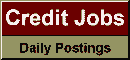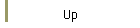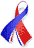DefaultRisk.com the web's biggest credit risk modeling resource.Export citation to:- HTML- Text (plain)- BibTeX- RIS- ReDIF

On Default Correlation: A copula function approach

by David X. Li of the RiskMetrics Group

September 1999

Abstract: This paper studies the problem of default correlation. We first introduce a random variable called "time-until-default" to denote the survival time of each defaultable entity or financial instrument, and define the default correlation between two credit risks as the correlation coefficient between their survival times. Then we argue why a copula function approach should be used to specify the joint distribution of survival times after marginal distributions of survival times are derived from market information, such as risky bond prices or asset swap spreads. The definition and some basic properties of copula functions are given. We show that the current CreditMetrics approach to default correlation through asset correlation is equivalent to using a normal copula function. Finally, we give some numerical examples to illustrate the use of copula functions in the valuation of some credit derivatives, such as credit default swaps and first-to-default contracts.

Published in: Journal of Fixed Income, Vol. 9, No. 4, (March 2000), pp. 43-54.

Books Referenced in this paper:  (what is this?)